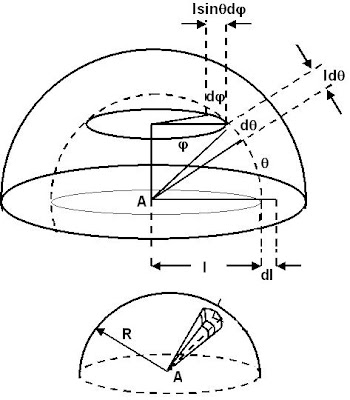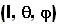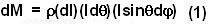## Sunday, September 14, 2008

### Irodov Problem 1.213Consider an infinitesimally small piece of the hemisphere located at polar coordinateswith the origin as the center of the hemisphere. The mass of this infinitesimally small piece is given byThe gravitational potential energy of mass m due this small mass dM will be,The total gravitational potential energy of the point mass at A can be obtained by integrating (2) over the entire volume of the hemisphere given by,The work done by gravity on the particle as it moves to infinity is exactly the gravitational potential energy of the mass at A.